## 深度学习：感知机和人工神经网络

9010 字 · 249 阅读 · 2023 年 06 月 11 日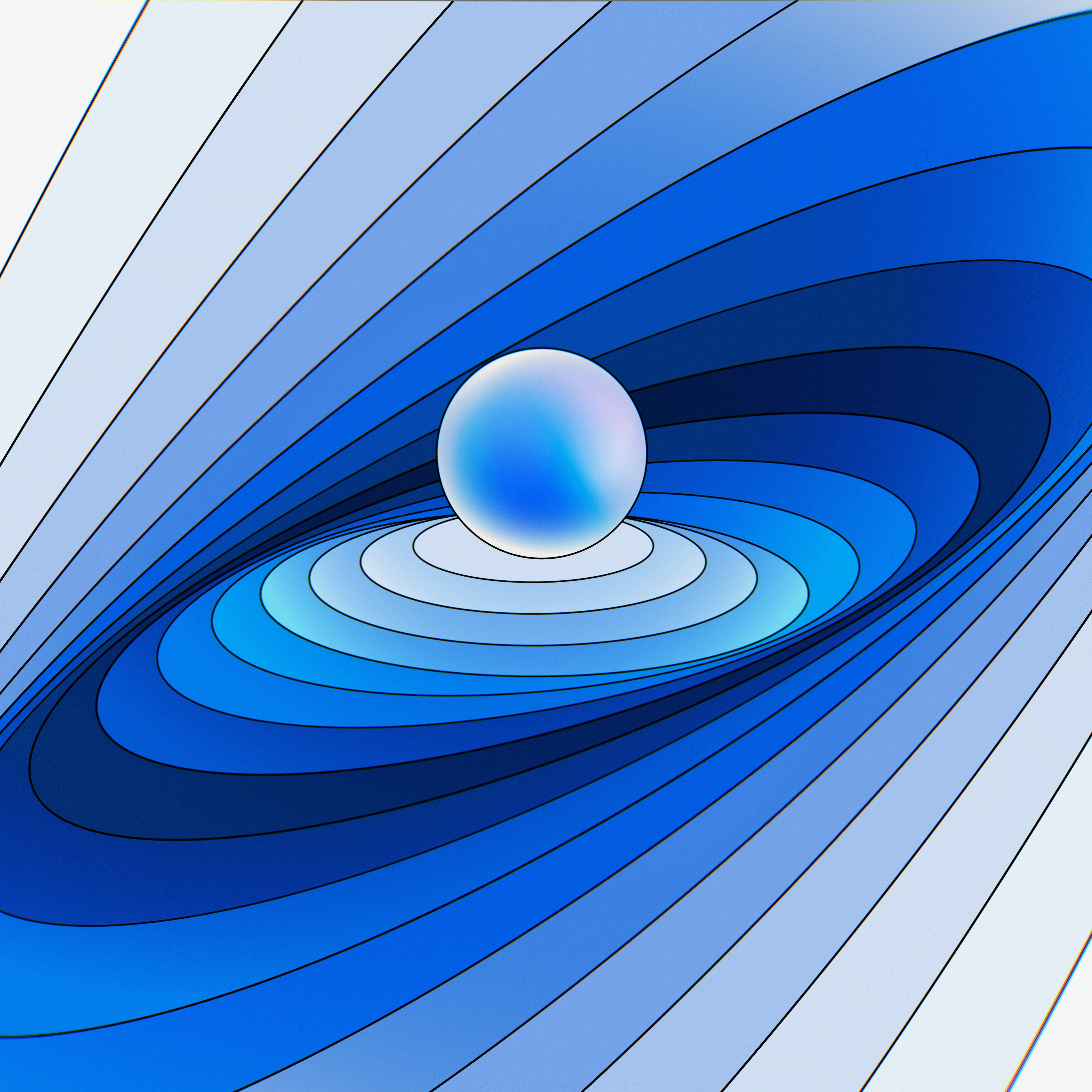• 感知机的推导过程
• 随机梯度下降法
• 多层感知机与人工神经网络
• 反向传播算法
• 实现人工神经网络

### 感知机的推导过程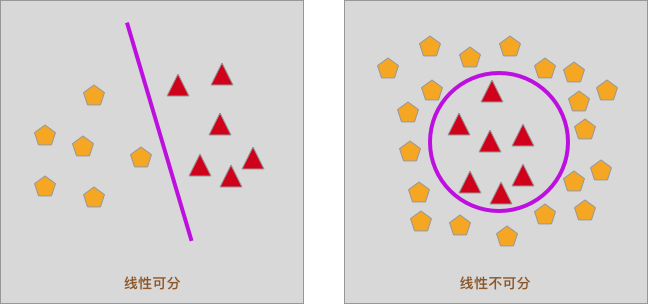$$f(x) = w_1x_1+w_2x_2+ \cdots +w_nx_n + b = WX+b \tag{1}$$

$$\operatorname{sign}(x)=\left\{\begin{array}{ll}{+1,} & {\text { if } x \geq 0} \\ {-1,} & {\text { if } x<0}\end{array}\right. \tag{2}$$

Sign 函数又被称之为符号函数，它的函数值只有 2 个。即当自变量 $x \geq 0$ 时，因变量为 1。同理，当 $x < 0$ 时，因变量为 -1。函数图像如下：$$f(x) = sign(W*x +b) \tag{3}$$

### 感知机计算流程图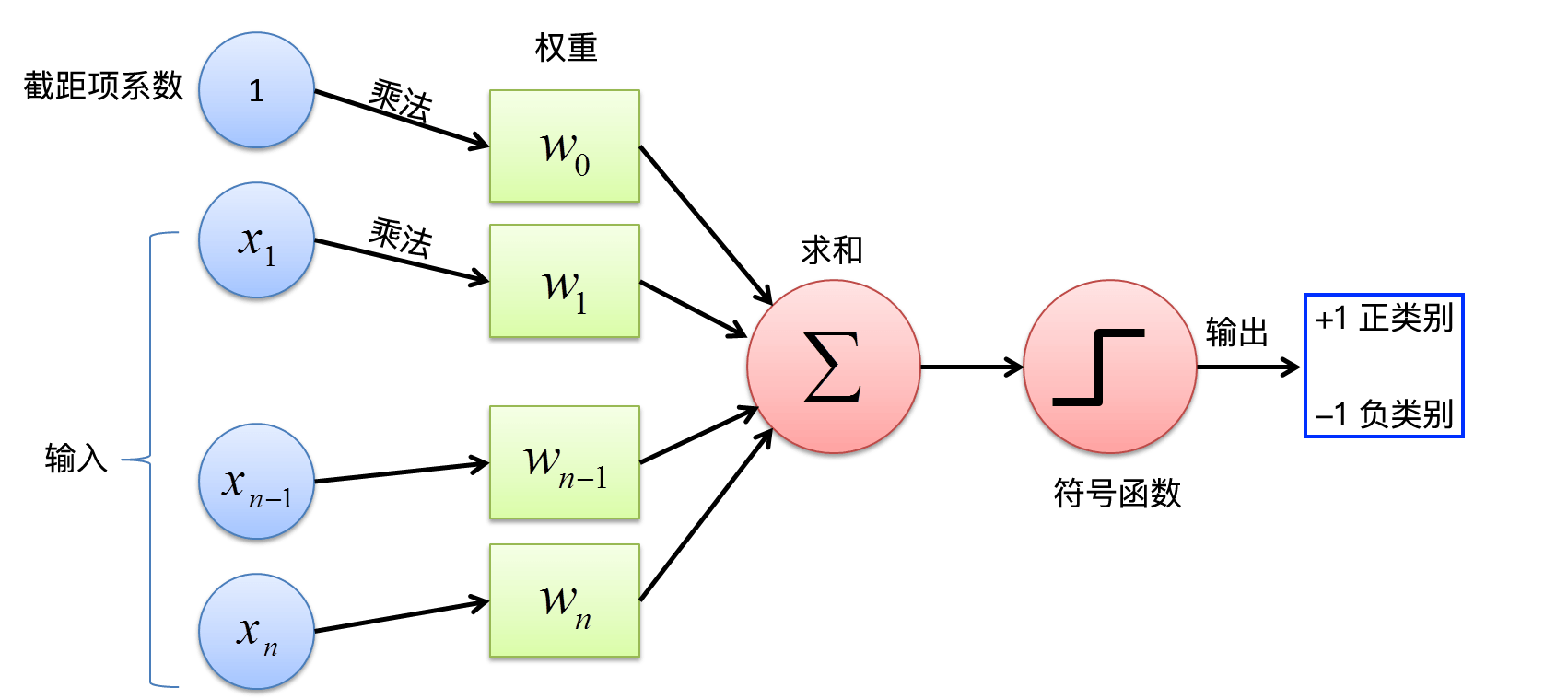### 感知机的损失函数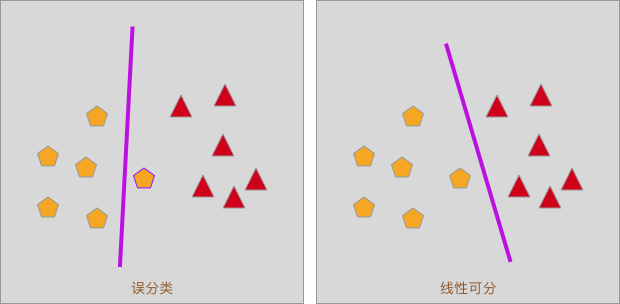#### 点到直线的距离

$$d= \dfrac{1}{\parallel W\parallel}|W*x_{0}+b| \tag{4}$$

$$• y_i(W * x_{i}+b)>0 \tag{5}$$ 将误分类点的坐标 $(x_i,y_i)$ 代入公式 $(4)$，通过 $W * x_i + b$ 与 $0$ 的大小比较去除绝对值，便可得到误分类点到分割线（面）的距离为：$$d=-\dfrac{1}{\parallel W\parallel}y_i(W*x_{i}+b) \tag{6}$$ 于是，假设所有误分类点的集合为 $M$，全部误分类点到分割线（面）的距离就为：$$-\dfrac{1}{\parallel W\parallel}\sum_{x_i\epsilon M} y_i(W*x_{i}+b) \tag{7}$$ 最后得到感知机的损失函数为：$$J(W,b) = - \sum_{x_i\epsilon M} y_i(W*x_{i}+b) \tag{8}$$

### 随机梯度下降法

$$min_{W,b} J(W,b) = - \sum_{x_i\epsilon M} y_i(W*x_{i}+b) \tag{9}$$

$$\frac{\partial J(W,b)}{\partial W} = - \sum_{x_i\epsilon M}y_ix_i \tag{10a}$$
$$\frac{\partial J(W,b)}{\partial b} = - \sum_{x_i\epsilon M}y_i \tag{10b}$$

$$W \leftarrow W + \lambda y_ix_i \tag{11a}$$
$$b \leftarrow b + \lambda y_i \tag{11b}$$

from sklearn.utils import shuffle

def perceptron_sgd(X, Y, alpha, epochs):
"""
参数:
X -- 自变量数据矩阵
Y -- 因变量数据矩阵
alpha -- lamda 参数
epochs -- 迭代次数

返回:
w -- 权重系数
b -- 截距项
"""
# 感知机随机梯度下降算法实现
w = np.zeros(len(X))  # 初始化参数为 0
b = np.zeros(1)

for t in range(epochs):  # 迭代
# 每一次迭代循环打乱训练样本
# X, Y = shuffle(X, Y)
for i, x in enumerate(X):
if ((np.dot(X[i], w)+b)*Y[i]) <= 0:  # 判断条件
w = w + alpha*X[i]*Y[i]  # 更新参数
b = b + alpha*Y[i]

return w, b


#### 小贴士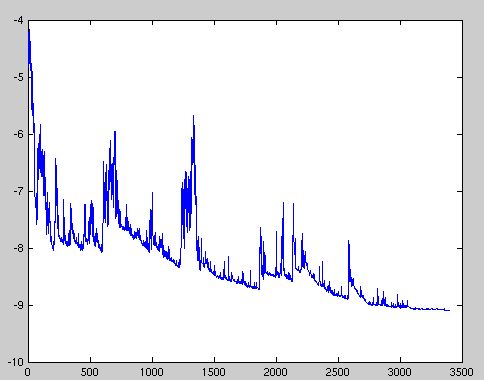### 感知机分类实例

#### 示例数据集

import pandas as pd



from matplotlib import pyplot as plt
%matplotlib inline

# 绘制数据集
plt.figure(figsize=(10, 6))
plt.scatter(df['X0'], df['X1'], c=df['Y'])


#### 感知机训练

import numpy as np

X = df[['X0', 'X1']].values
Y = df['Y'].values

alpha = 0.1
epochs = 150

perceptron_sgd(X, Y, alpha, epochs)


$$f(x)=4.93 * x_{1}-6.98 * x_{2}-3.3 \tag{12}$$

L = perceptron_sgd(X, Y, alpha, epochs)
w1 = L
w2 = L
b = L

z = np.dot(X, np.array([w1, w2]).T) + b
np.sign(z)


from sklearn.metrics import accuracy_score

accuracy_score(Y, np.sign(z))


#### 绘制决策边界线

# 绘制轮廓线图，不需要掌握
plt.figure(figsize=(10, 6))
plt.scatter(df['X0'], df['X1'], c=df['Y'])

x1_min, x1_max = df['X0'].min(), df['X0'].max(),
x2_min, x2_max = df['X1'].min(), df['X1'].max(),

xx1, xx2 = np.meshgrid(np.linspace(x1_min, x1_max),
np.linspace(x2_min, x2_max))
grid = np.c_[xx1.ravel(), xx2.ravel()]

probs = (np.dot(grid, np.array(
[L, L]).T) + L).reshape(xx1.shape)
plt.contour(xx1, xx2, probs, , linewidths=1, colors='red')


#### 绘制损失函数变换曲线

def perceptron_loss(X, Y, alpha, epochs):
"""
参数:
X -- 自变量数据矩阵
Y -- 因变量数据矩阵
alpha -- lamda 参数
epochs -- 迭代次数

返回:
loss_list -- 每次迭代损失函数值列表
"""
# 计算每次迭代后的损失函数值
w = np.zeros(len(X))  # 初始化参数为 0
b = np.zeros(1)
loss_list = []

for t in range(epochs):  # 迭代
loss_init = 0
for i, x in enumerate(X):
# 每一次迭代循环打乱训练样本
# X, Y = shuffle(X, Y)
if ((np.dot(X[i], w)+b)*Y[i]) <= 0:  # 判断条件
loss_init += (((np.dot(X[i], w)+b)*Y[i]))
w = w + alpha*X[i]*Y[i]  # 更新参数
b = b + alpha*Y[i]
loss_list.append(loss_init * -1)

return loss_list

loss_list = perceptron_loss(X, Y, alpha, epochs)

plt.figure(figsize=(10, 6))
plt.plot([i for i in range(len(loss_list))], loss_list)
plt.xlabel("Learning rate {}, Epochs {}".format(alpha, epochs))
plt.ylabel("Loss function")alpha = 0.05  # 减小学习率
epochs = 1000  # 增加迭代次数

loss_list = perceptron_loss(X, Y, alpha, epochs)

plt.figure(figsize=(10, 6))
plt.plot([i for i in range(len(loss_list))], loss_list)
plt.xlabel("Learning rate {}, Epochs {}".format(alpha, epochs))
plt.ylabel("Loss function")


L = perceptron_sgd(X, Y, alpha, epochs)
z = np.dot(X, L.T) + L
accuracy_score(Y, np.sign(z))


### 多层感知机与人工神经网络

#### 小贴士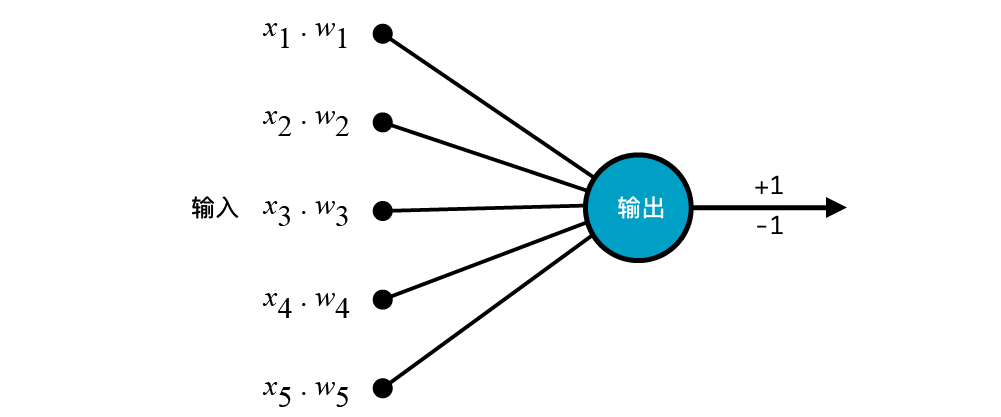### 激活函数

$$f(x) = w_1x_1+w_2x_2+ \cdots +w_nx_n + b = WX+b \tag{13}$$
• 对于逻辑回归而言，我们是采用了 $sigmoid$ 函数将 $f(x)$ 转换为概率，最终实现二分类。
• 对于感知机而言，我们是采用了 $sign$ 函数将 $f(x)$ 转换为 -1 和 +1 最终实现二分类。
• 对于多层感知机而言，具有多层神经网络结构，在 $f(x)$ 的处理方式上，一般会有更多的操作。

#### $sigmoid$ 函数

$sigmoid$ 函数应该已经非常熟悉了吧，它的公式如下：

$$sigmoid(x)=\frac{1}{1+e^{-x}} \tag{14}$$

$sigmoid$ 函数的图像呈 S 型，函数值介于 $(0, 1)$ 之间：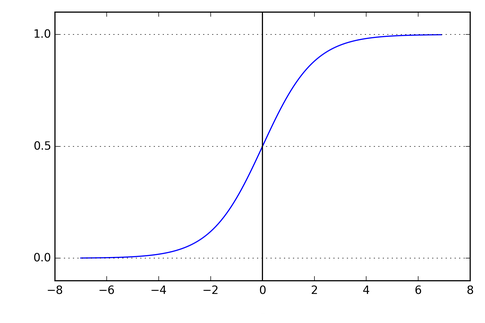#### $Tanh$ 函数

$Tanh$ 函数与 $sigmoid$ 函数的图像很相似，都呈 S 型，只不过 $Tanh$ 函数值介于 $(-1, 1)$ 之间，公式如下：

$$tanh(x)=\frac{1-e^{-2x}}{1+e^{-2x}}\tag{15}$$#### $ReLU$ 函数

$ReLU$ 函数全称叫做 Rectified Linear Unit，也就是修正线性单元，公式如下：

$$ReLU(x) = max(0,x)\tag{16}$$

$ReLU$ 有很多优点，比如收敛速度会较快且不容易出现梯度消失。由于这次实验不会用到，我们之后再说。$ReLU$ 的图像如下：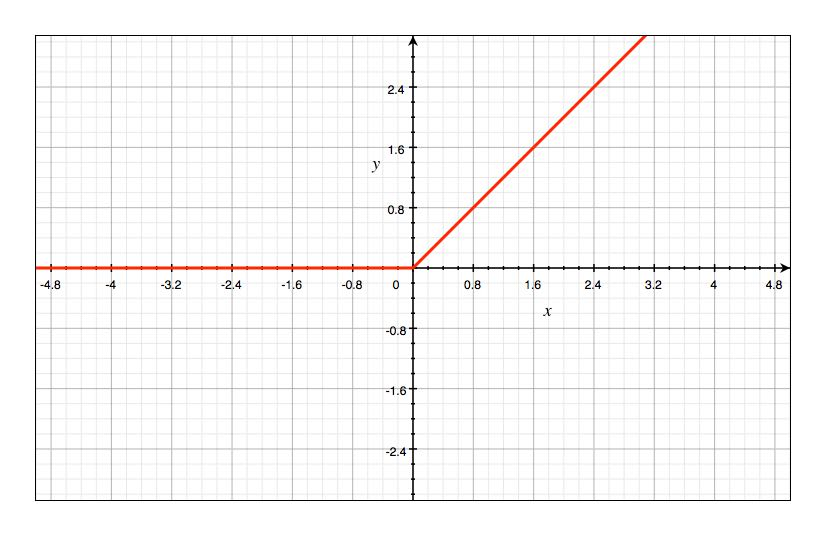#### 激活函数的作用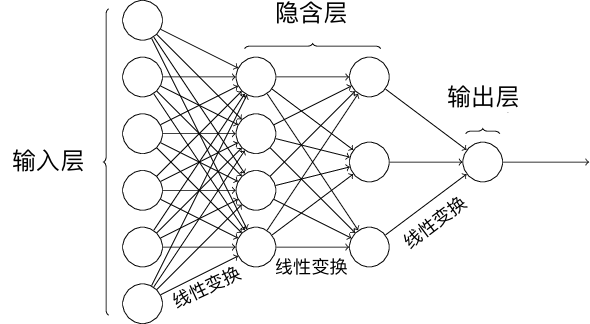### 反向传播算法（BP）直观认识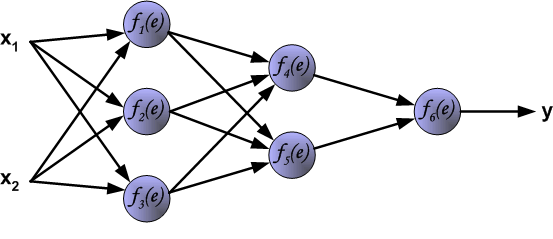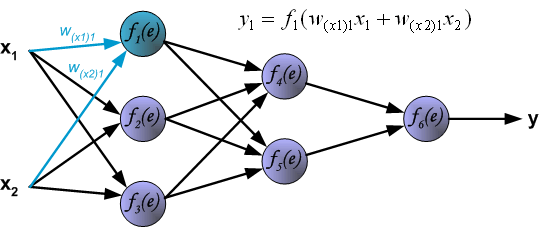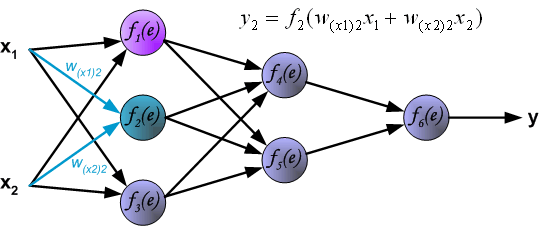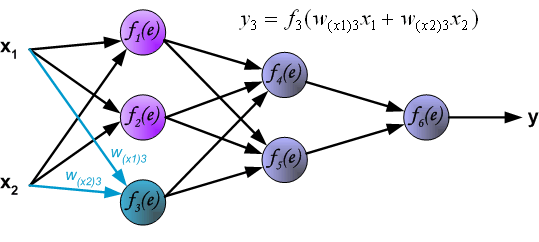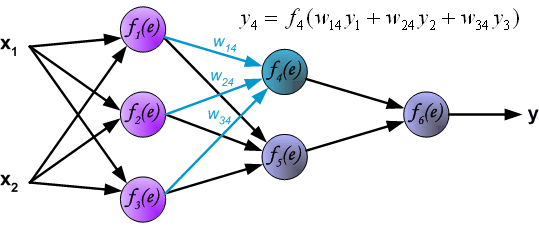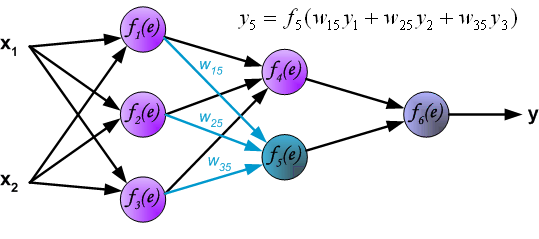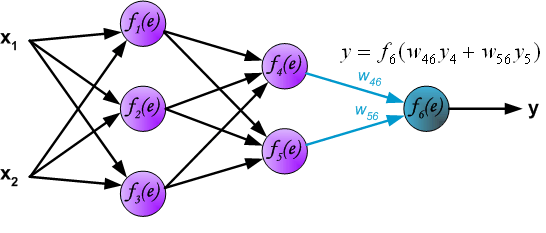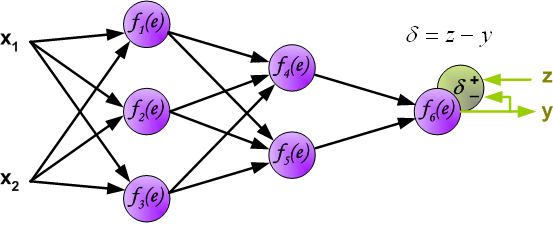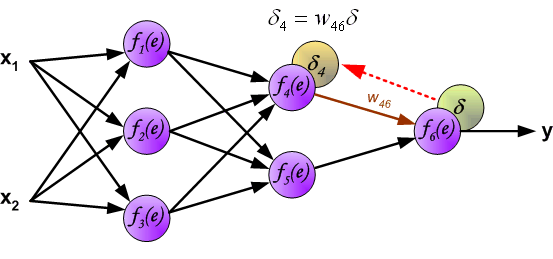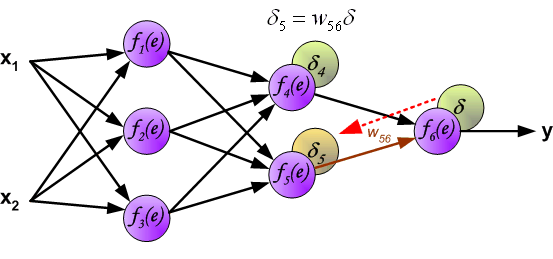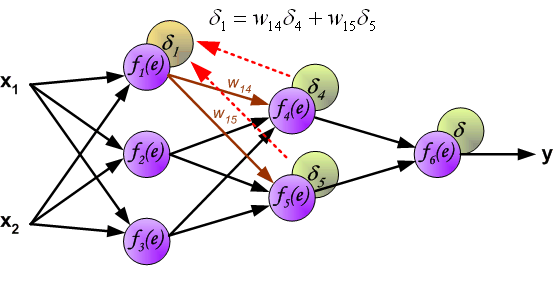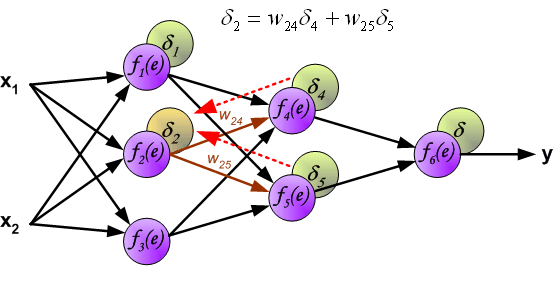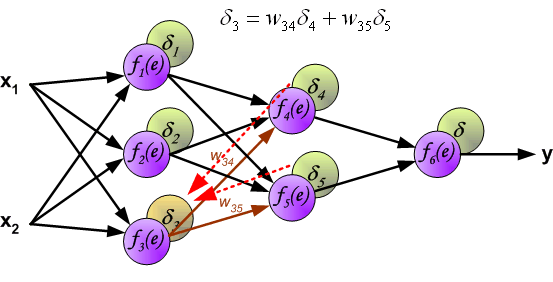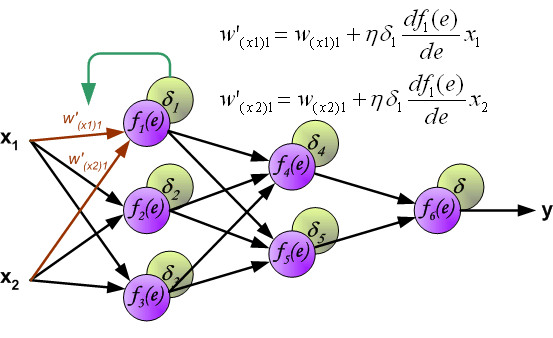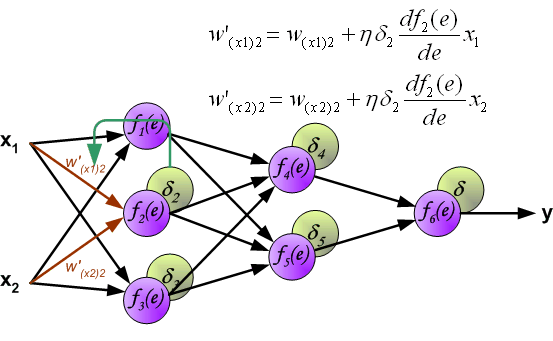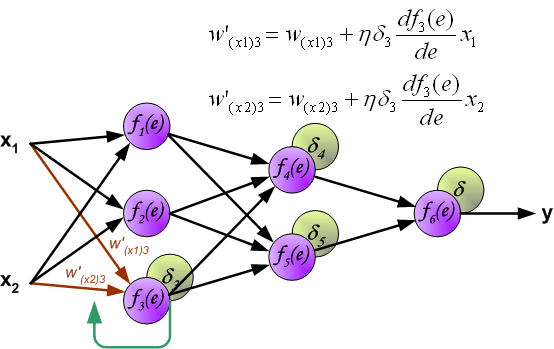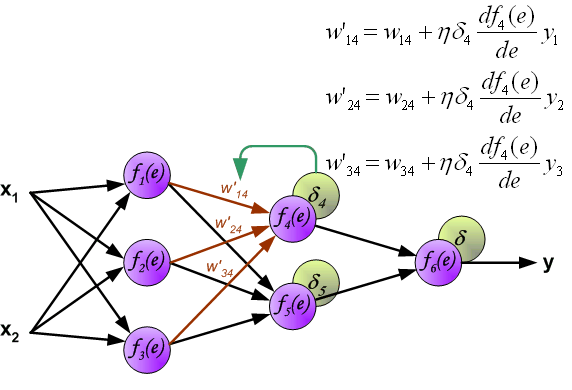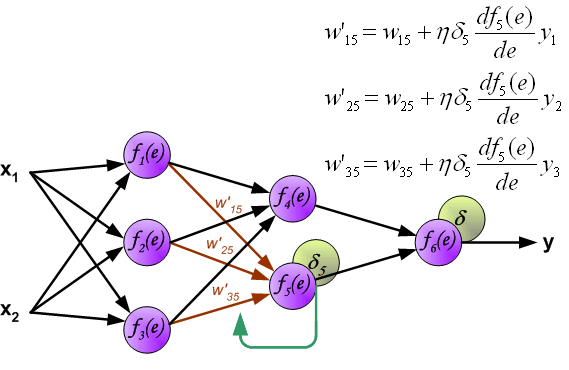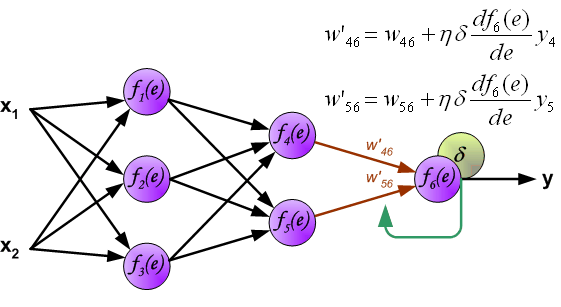### Python 实现人工神经网络

#### 定义神经网络结构$$\mathit{sigmoid}(x) = \frac{1}{1+e^{-x}} \tag{17a}$$

$$\Delta \mathit{sigmoid}(x) = \mathit{sigmoid}(x)(1 - \mathit{sigmoid}(x)) \tag{17b}$$

def sigmoid(x):
# sigmoid 函数
return 1 / (1 + np.exp(-x))

def sigmoid_derivative(x):
# sigmoid 函数求导
return sigmoid(x) * (1 - sigmoid(x))


#### 前向传播

• $Z$ 表示隐含层输出，$Y$ 则为输出层最终输出。
• $w_{ij}$ 表示从第 $i$ 层的第 $j$ 个权重。

$$X=\left[ \begin{array}{ll}{x_{1}} & {x_{2}}\end{array}\right] \tag{18}$$
$$W_{1}=\left[ \begin{array}{lll}{w_{11}} & {w_{12}} & {w_{13}} \\ {w_{14}} & {w_{15}} & {w_{16}}\end{array}\right] \tag{19}$$
$$W_{2}=\left[ \begin{array}{c}{w_{21}} \\ {w_{22}} \\ {w_{23}}\end{array}\right] \tag{20}$$

$$Z = \mathit{sigmoid}(X \cdot W_{1}) \tag{21}$$

$$Y = \mathit{sigmoid}(Z \cdot W_{2}) \tag{22}$$

# 示例样本
X = np.array([[1, 1]])
y = np.array([])

X, y


W1 = np.random.rand(2, 3)
W2 = np.random.rand(3, 1)

W1, W2


input_layer = X  # 输入层
hidden_layer = sigmoid(np.dot(input_layer, W1))  # 隐含层，公式 20
output_layer = sigmoid(np.dot(hidden_layer, W2))  # 输出层，公式 22

output_layer


#### 反向传播

$$Loss(y, Y) = \sum (y - Y)^2 \tag{23}$$

$$\frac{\partial Loss(y, Y)}{\partial{W_2}} = \frac{\partial Loss(y, Y)}{\partial{Y}} \frac{\partial Y}{\partial{W_2}}\tag{24a}$$
$$\frac{\partial Loss(y, Y)}{\partial{W_2}} = 2(Y-y) * \Delta \mathit{sigmoid}(Z \cdot W_2) \cdot Z\tag{24b}$$

$$\frac{\partial Loss(y, Y)}{\partial{W_1}} = \frac{\partial Loss(y, Y)}{\partial{Y}} \frac{\partial Y }{\partial{Z}} \frac{\partial Z}{\partial{W_1}} \tag{25a}$$
$$\frac{\partial Loss(y, Y)}{\partial{W_1}} = 2(Y-y) * \Delta \mathit{sigmoid}(Z \cdot W_2) \cdot W_2 * \Delta \mathit{sigmoid}(X \cdot W_1) \cdot X \tag{25b}$$

# 公式 24
d_W2 = np.dot(hidden_layer.T, (2 * (output_layer - y) *
sigmoid_derivative(np.dot(hidden_layer, W2))))

# 公式 25
d_W1 = np.dot(input_layer.T, (
np.dot(2 * (output_layer - y) * sigmoid_derivative(
np.dot(hidden_layer, W2)), W2.T) * sigmoid_derivative(np.dot(input_layer, W1))))

d_W2, d_W1


# 梯度下降更新权重, 学习率为 0.05

W1 -= 0.05 * d_W1  # 如果上面是 y - output_layer，则改成 +=
W2 -= 0.05 * d_W2

W2, W1


# 示例神经网络完整实现
class NeuralNetwork:

# 初始化参数
def __init__(self, X, y, lr):
self.input_layer = X
self.W1 = np.random.rand(self.input_layer.shape, 3)
self.W2 = np.random.rand(3, 1)
self.y = y
self.lr = lr
self.output_layer = np.zeros(self.y.shape)

# 前向传播
def forward(self):
self.hidden_layer = sigmoid(np.dot(self.input_layer, self.W1))
self.output_layer = sigmoid(np.dot(self.hidden_layer, self.W2))

# 反向传播
def backward(self):
d_W2 = np.dot(self.hidden_layer.T, (2 * (self.output_layer - self.y) *
sigmoid_derivative(np.dot(self.hidden_layer, self.W2))))

d_W1 = np.dot(self.input_layer.T, (
np.dot(2 * (self.output_layer - self.y) * sigmoid_derivative(
np.dot(self.hidden_layer, self.W2)), self.W2.T) * sigmoid_derivative(
np.dot(self.input_layer, self.W1))))

# 参数更新
self.W1 -= self.lr * d_W1
self.W2 -= self.lr * d_W2


X = df[['X0', 'X1']].values  # 输入值
y = df[['Y']].values # 真实 y


nn = NeuralNetwork(X, y, lr=0.001)  # 定义模型
loss_list = []  # 存放损失数值变化

for i in range(100):
nn.forward()  # 前向传播
nn.backward()  # 反向传播
loss = np.sum((y - nn.output_layer)**2)  # 计算平方损失
loss_list.append(loss)

print("final loss:", loss)
plt.plot(loss_list)  # 绘制 loss 曲线变化图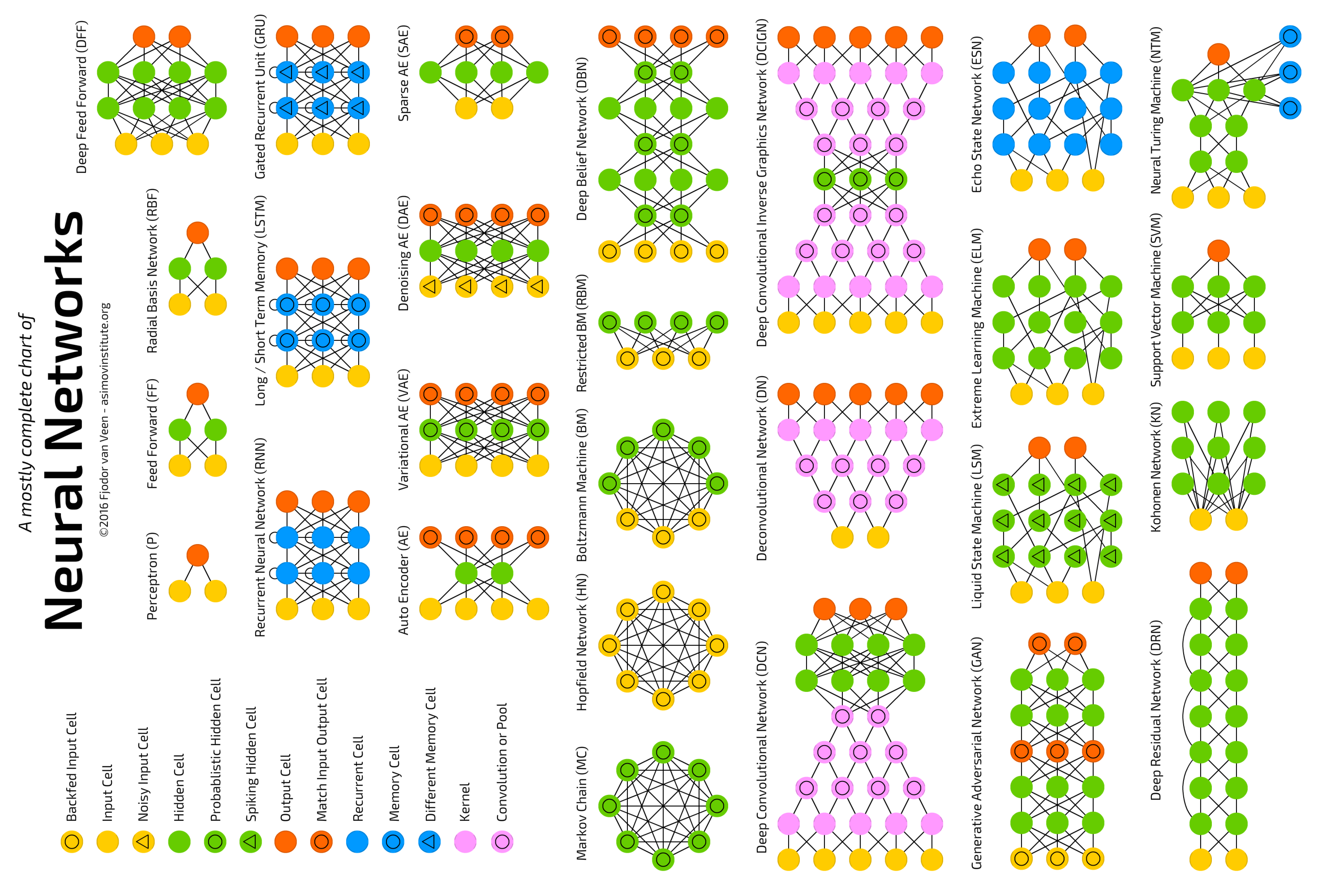### 系列文章•